# How To Solve Quadratic Equations (4 Methods To Know)

Quadratic equations come up often in mathematics and physics, and it is vital to know how to solve them.  Luckily, there are several ways to do it.

So, how do you solve quadratic equations?  You can solve quadratic equations by graphing, factoring, completing the square, & the quadratic formula. Graphing gives a good visual, but it is hard to find values of x from a graph with no equation. If factoring is hard, the quadratic formula (a shortcut for completing the square) helps.

Of course, the quadratic formula will work for any quadratic equation you choose.  However, it might be easier to factor in some cases to avoid radicals and fractions in the quadratic formula.

In this article, we’ll talk about the four methods you can use to solve a quadratic equation and give some examples for each one.  We’ll also take a closer look at how these methods are connected to each other.

Let’s get started.

## How To Solve Quadratic Equations

Here are four methods you can use to solve a quadratic equation:

• Graphing – this is a good visual method if you have the vertex form of a parabola or if you have a parabola-like curve from a data set.  If you graph the quadratic function f(x) = ax2 + bx + c, you can find out where it intersects the x-axis.  The only drawback is that it can be difficult to find exact values of x.  Also, the graph will not intersect the x-axis if the solutions are complex (in the case of a negative discriminant).
• Factoring – this method is helpful in some cases to avoid the work of graphing, completing the square, or using the quadratic formula.  However, some quadratics are difficult to factor, and the quadratic formula would be more helpful in those cases.
• Complete the Square this method is a long one, but it works.  The quadratic formula is a shortcut for this method.  We also use the method of completing the square to put certain circle equations into the proper form.
• Quadratic Formula – this method always works.  It is derived from using the previous method (complete the square) on a general quadratic in standard form: ax2 + bx + c = 0.  The only drawback is that the calculations can become tedious, as they involve multiplication, addition, radicals, and division (fractions).

Here is how these methods are connected to one another:

• When the method of completing the square is used on the general quadratic equation in standard form, ax2 + bx + c = 0, we get the quadratic formula as a result.
• When we use the quadratic formula on a quadratic equation, we get two solutions to the equation: r and s.  These solutions may be distinct and real (positive discriminant), double real (zero discriminant), or complex conjugates (negative discriminant).
• If we use the coefficient ‘a’ together with the solutions r and s, we can write the factored form of the quadratic equation as a(x – r)(x – s) = 0.
• If we take the average of the two solutions r and s, we can find the x-coordinate of the parabola’s vertex (that is, the axis of symmetry).  If r and s are real, we also have x-intercepts for the parabola, which makes it easier to graph.

Let’s take a look at some examples of each method, starting with graphing.

When Does A Quadratic Have No Solut...
When Does A Quadratic Have No Solution?

### How To Solve Quadratic Equations By Graphing

To solve a quadratic equation by graphing, all we really need to do is find out where the zeros are (the points where the graph intersects the x-axis).

#### Example: Solving A Quadratic Equation By Graphing

Consider the following parabola (the graph of a quadratic):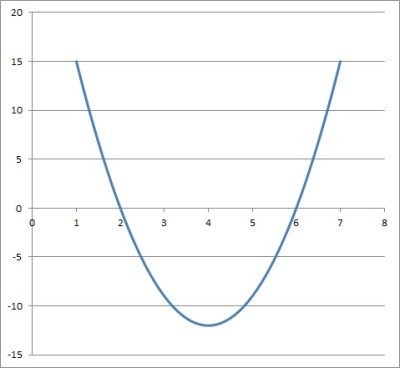We can sometimes find the solutions of a quadratic equation by graphing and finding the x-intercepts (zeros).

We can see from the graph that the parabola intersects the x-axis (the line y = 0) at x = 2 and x= 6.  At this point, we know that the solutions of the equation are x = 2 and x = 6.

However, we can find the quadratic equation as well.  First, the quadratic factored form looks like this:

• y = a(x – r)(x – s)
• y = a(x – 2)(x – 6)  [r = 2 and s = 6 are the two solutions of the quadratic equation]

Now we just need to find the value of a.  To do that, we can use the vertex of the parabola.

We know that its x-coordinate will be the average of the zeros x = 2 ad x = 6.  This gives us an x-coordinate of x = 4 for the vertex.

Looking at the graph again, we can see that the vertex is indeed at x = 4, with a value of y = -12.  We will use this value in the quadratic to solve for a:

• y = a(x – 2)(x – 6)
• -12 = a(4 – 2)(4 – 6)
• -12 = a(2)(-2)
• -12 = -4a
• 3 = a

So the value of a is 3, and the quadratic factored form is:

• y = a(x – 2)(x – 6)
• y = 3(x – 2)(x – 6)  [substitute a = 3]
• y = 3(x2 – 8x + 12)  [FOIL]
• y = 3x2 – 24x + 36) [distribute the 3 through parentheses]

Now we know the solutions to the quadratic equation, along with the standard and factored forms.

### How To Solve Quadratic Equations By Factoring

There are lots of cases when it is easier to factor a quadratic equation than to graph, complete the square, or use the quadratic formula.

However, it takes a little practice to get good at spotting when you can factor a quadratic easily.  Let’s take a look at some examples.

#### Example 1: Solving A Quadratic Equation By Factoring

Consider the following quadratic equation:

• x2 – 16 = 0

We can see that the left side is a difference of squares: x2 – 42.  Remember that the formula to factor a difference of squares is:

• A2 – B2 = (A + B)(A – B)

Using this formula here with A = x and B = 4, we get:

• A2 – B2 = (A + B)(A – B)
• x2 – 42 = (x + 4)(x – 4)
• x2 – 16 = (x + 4)(x – 4)

Now we can rewrite the original quadratic equation as:

• x2 – 16 = 0
• (x + 4)(x – 4) = 0

The zero product property tells us that either

• x + 4 = 0

or

• x – 4 = 0

This means that x = 4 and x = -4 are both solutions to the quadratic equation.  You can see this in the graph below.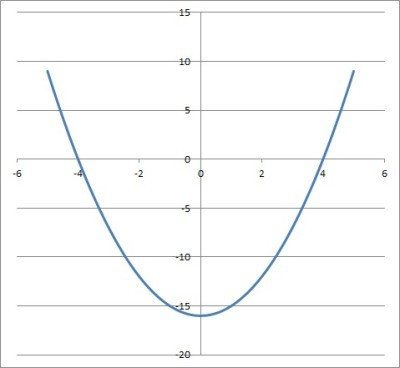The graph of the quadratic y = x2 – 16. It has zeros at x = 4 and x = -4, which are the solutions to the corresponding quadratic equation.

#### Example 2: Solving A Quadratic Equation By Factoring

Sometimes we need to factor out a GCF (greatest common factor) before we can use another factoring method.

Consider the following quadratic equation:

• 4x2 – 36 = 0

We can factor out a GCF of 4 from both of the terms on the left side, which leaves us with:

• 4(x2 – 9) = 0

We can see that the left side of the expression in parentheses is a difference of squares: x2 – 32

Using the difference of squares formula from example 1 with A = x and B = 3, we get:

• A2 – B2 = (A + B)(A – B)
• x2 – 32 = (x + 3)(x – 3)
• x2 – 9 = (x + 3)(x – 3)

Now we can rewrite the original quadratic equation as:

• 4x2 – 36 = 0
• 4(x2 – 9) = 0
• 4(x+ 3)(x – 3) = 0

The zero product property tells us that either

• x + 3 = 0

or

• x – 3 = 0

This means that x = 3 and x = -3 are both solutions to the quadratic equation.  You can see this in the graph below.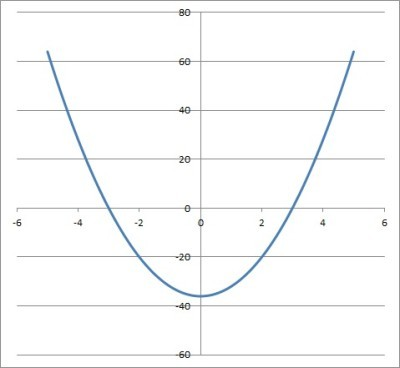The graph of the quadratic y = 4x2 – 36. It has zeros at x = 3 and x = -3, which are the solutions to the corresponding quadratic equation.

#### Example 3: Solving A Quadratic Equation By Factoring

Consider the following quadratic equation:

• 5x2 – 10x = 0

We can factor out a GCF of 5x from both terms on the left to get:

• 5x(x – 2) = 0

The zero product property tells us that either

• x = 0

or

• x – 2 = 0

This means that x = 0 and x = 2 are both solutions to the quadratic equation.  You can see this in the graph below.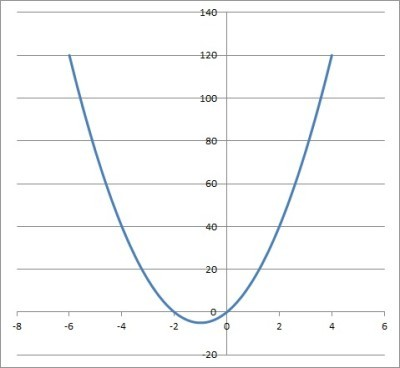The graph of the quadratic y = 5x2 – 10x. It has zeros at x = 0 and x = 2, which are the solutions to the corresponding quadratic equation.

#### Example 4: Solving A Quadratic Equation By Factoring

Consider the following quadratic equation:

• x2 – 10x + 25 = 0

We can see that the constant term is a perfect square: 25 = 52.  We can also see that the linear coefficient is -10, which is -2*5.

This tells us that we have a perfect square trinomial.  The formula for factoring a perfect square trinomial is:

• x2 – 2Ax + A2 = (x – A)2

or

• x2 – 2Ax + A2 = (x – A)(x – A)

Using the perfect square trinomial formula here with A = 5, we get:

• x2 – 2Ax + A2 = (x – A)(x – A)
• x2 – 2(5)x  + 52 = (x – 5)(x – 5)
• x2 – 10x  + 25 = (x – 5)(x – 5)

Now we can rewrite the original quadratic equation as:

• x2 – 10x + 25= 0
• (x – 5)(x – 5) = 0

The zero product property tells us that

• x – 5 = 0

This means that x = 5 is a double root or repeated solution to the quadratic equation.  You can see this in the graph below.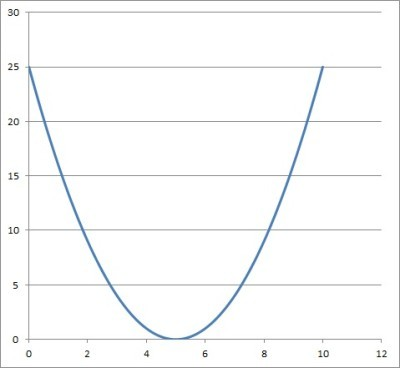The graph of the quadratic y = x2 – 10x + 25. It has a zero at x = 5 (a double root), which is the solution to the corresponding quadratic equation.

#### Example 5: Solving A Quadratic Equation By Factoring

Consider the following quadratic equation:

• x2 – 7x + 6 = 0

We can see that the constant term can only factor as 6 = 1*6 or 6 = (-1)*(-6).  Since we want a negative linear term, we choose the negative factors.

Since -1 + -6 = -7, we have found the right pair of values to use: -1 and -6.  Applying these, we get:

• x2 – 7x + 6 = 0
• (x – 1)(x – 6) = 0

The zero product property tells us that either

• x – 1 = 0

or

• x – 6 = 0

This means that x = 1 and x = 6 are both solutions to the quadratic equation.  You can see this in the graph below.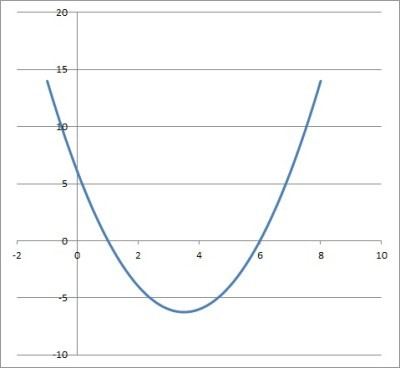The graph of the quadratic y = x2 – 7x + 6. It has zeros at x = 1 and x = 6, which are the solutions to the corresponding quadratic equation.

### How To Solve Quadratic Equations By Completing The Square

Completing the square is another method we can use to solve a quadratic equation.  This method is used to derive the quadratic formula, and it can be helpful for converting circle equations into a form that we can graph easily.

#### Example 1: Solving A Quadratic Equation By Completing The Square

Consider the following quadratic equation:

• x2 – 6x + 2 = 0

We cannot factor as we did earlier, but we can complete the square to solve.

First, we rearrange the equation so that it has the form x2 + Bx = C.  Here, this means we subtract 2 from both sides to get:

• x2 – 6x = -2

Remember that if we have x2 + Bx = C, then we take half of B, square it, and then add that amount to both sides of the equation (that is, add B2 / 4 to both sides).

Here, we have B = -6, so we add B2 / 4 = (-6)2 / 4 = 36 / 4 = 9 to both sides of the equation:

• x2 – 6x + 9 = -2 + 9
• x2 – 6x + 9 = 7

Now we have a perfect square trinomial on the left side, with A = 3.  This factors as:

• (x – 3)2 = 7

Now we can take the square root of both sides to get:

• √(x – 3)2 = √7
• x – 3 = √7

The two solutions are x = 3 + √7 and x = 3 – √7.  You can see this in the graph below.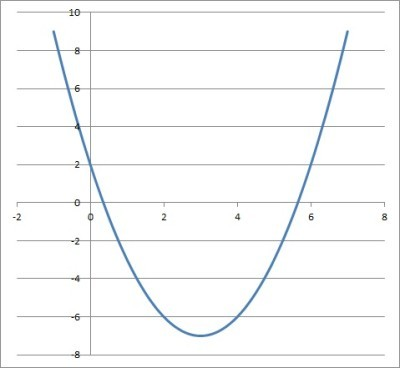The graph of the quadratic y = x2 – 6x + 2. It has zeros at x = 3 + √7 and x = 3 – √7, which are the solutions of the corresponding quadratic equation.

#### Example 2: Solving A Quadratic Equation By Completing The Square

Consider the following quadratic equation:

• x2 – 4x + 40 = 0

We cannot factor as we did earlier, but we can complete the square to solve.

First, we rearrange the equation so that it has the form x2 + Bx = C.  Here, this means we subtract 40 from both sides to get:

• x2 – 4x = -40

Again, if we have x2 + Bx = C, then we take half of B, square it, and then add that amount to both sides of the equation (that is, add B2 / 4 to both sides).

Here, we have B = -4, so we add B2 / 4 = (-4)2 / 4 = 16 / 4 = 4 to both sides of the equation:

• x2 – 4x + 4 = -40 + 4
• x2 – 4x + 4 = -36

Now we have a perfect square trinomial on the left side, with A = 2.  This factors as:

• (x – 2)2 = -36

Now we can take the square root of both sides to get:

• √(x – 2)2 = √(-36)
• x – 2 = i√6

where i = √-1.

The two solutions are x = 2 + i√6 and x = 2 – i√6.  You can see the graph of this quadratic below (since the roots are complex, they do not appear on the graph).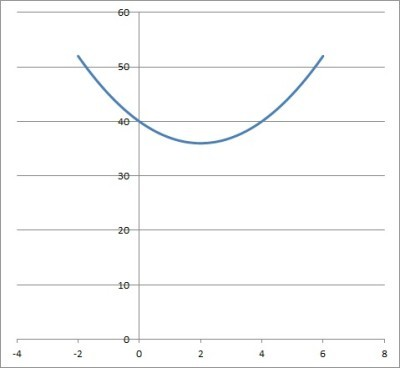The graph of the quadratic y = x2 – 4x + 40. It has zeros at x = 2 + i√6 and x = 2 – i√6, which are the solutions of the corresponding quadratic equation (these solutions are complex, so they do not appear on the graph).

### How To Solve Quadratic Equations By The Quadratic Formula

The quadratic formula is a guaranteed method to solve any quadratic formula.  No matter how difficult it is to factor, the quadratic formula will always give us a solution.

However, we do have to contend with radicals and fractions when using the formula.  Remember that for a quadratic equation in standard form:

• ax2 + bx + c = 0

the quadratic formula is given by:

Let’s take a look at some examples of how to use it.

#### Example 1: Solving A Quadratic Equation By The Quadratic Formula

Consider the following quadratic equation:

• 2x2 – 16x + 30 = 0

Right away, we can make things easier by factoring out a GCF of 2 and dividing by 2 on both sides to get:

• 2x2 – 16x + 30 = 0
• 2(x2 – 8x + 15) = 0
• x2 – 8x + 15 = 0

This leaves us with smaller coefficients to work with, which makes our work in the quadratic formula a little easier.

This quadratic equation has a = 1, b = -8, and c = 15.  Substituting these values into the quadratic formula gives us:

• x = [-b +/- √(b2 – 4ac)] / 2a
• x = [-(-8) +/- √((-8)2 – 4(1)(15))] / 2(1)
• x = [8 +/- √(64 – 60)] / 2
• x = [8 +/- √4] / 2
• x = [8 +/- 2] / 2
• x = 10 / 2 or 6 / 2
• x = 5 or x = 3

This means that x = 3 and x = 5 are both solutions to the quadratic equation.  You can see this in the graph below.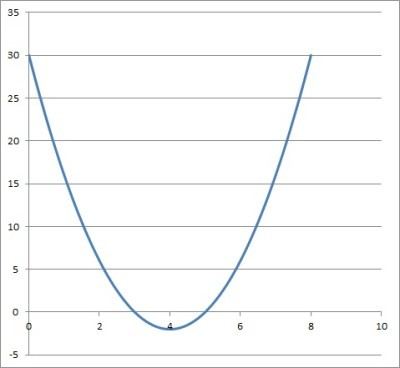The graph of the quadratic y = 2x2 – 16x + 30. It has zeros at x = 3 and x= 5, which are the solutions of the corresponding quadratic equation.

#### Example 2: Solving A Quadratic Equation By The Quadratic Formula

Consider the following quadratic equation:

• 3x2 + 6x + 11 = 0

This quadratic equation has a = 3, b = 7, and c = 12.  Substituting these values into the quadratic formula gives us:

• x = [-b +/- √(b2 – 4ac)] / 2a
• x = [-(6) +/- √((6)2 – 4(3)(11))] / 2(3)
• x = [6 +/- √(36 – 132)] / 6
• x = [6 +/- √(-96)] / 6
• x = [6 +/- 4i√(6)] / 6
• x = (6 + 4i√6) / 6 or x = (6 – 4i√6) / 6 or
• x = 1 + (2i√6) / 3) or x = 1 – (2i√6 / 3)

This means that x = 1 + (2i√6) / 3)  and x = 1 – (2i√6) / 3) are both solutions to the quadratic equation.  You can see the graph below (since the solutions are complex, they do not appear on the graph).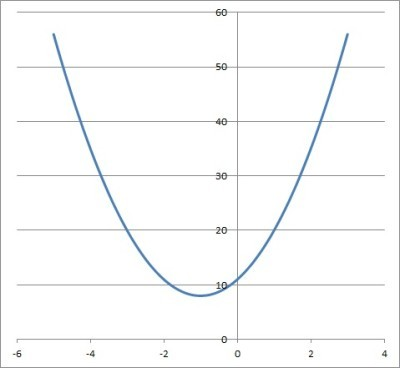The graph of the quadratic y = 2x2 – 16x + 30. It has zeros at x = 1 + (2i√6) / 3)  and x = 1 – (2i√6) / 3), which are the solutions of the corresponding quadratic equation (these solutions are complex, so they do not appear on the graph).

## Conclusion

Now you know about 4 methods you can use to solve quadratic equations.  You also know when it might be easier to use each one, and how the methods are connected to each other.

I hope you found this article helpful.  If so, please share it with someone who can use the information.

~Jonathon